# Projectile Motion Formula

The projectile motion is defined as the form of motion that is experienced by an object when it is projected into the air, which is subjected to the acceleration due to gravity. The projectile is the object while the path taken by the projectile is known as a trajectory. Let us know more about the projectile motion formula along with the projectile motion solved example.

## Projectile Motion Formula

Following are the formula of projectile motion which is also known as trajectory formula: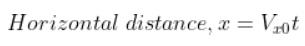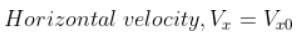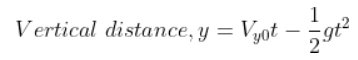Where,

• Vx  is the velocity (along the x-axis)
• Vxo is Initial velocity (along the x-axis)
• Vy is the velocity (along the y-axis)
• Vyo is initial velocity (along the y-axis)
• g is the acceleration due to gravity
• t is the time taken

Equations related to the projectile motion is given as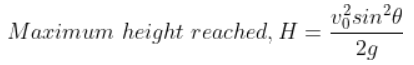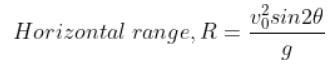Where

• Vo is the initial velocity
• sin θ is the component along the y-axis
• cos θ is the component along the x-axis

The formula of projectile motion is used to calculate the velocity, distance and time observed in the projectile motion of the object.

### Projectile Motion Solved Example

Problem 1: Jhonson is standing on the top of the building and John is standing down. If Jhonson tosses a ball with a velocity 30 m/s and at the angle of 70° then at the time 3s what height will the ball reach?

Given:

Vyo = 30 m/s

Δ t = 3s

The vertical velocity in the y-direction is expressed as

Vy = Vyo sin 70°

Vy = 30 sin 70

Vy = 23.22 m/s

See the video below, to understand projectile motion in a better way.Hope you have understood the projectile motion formula. To know more, stay tuned with BYJU’S.

## Frequently Asked Questions – FAQs

Q1

### Define projectile motion?

Projectile motion is the form of motion experienced by an object when it is projected into the air, which is subjected to acceleration due to gravity.
Q2

### What is the use of the projectile motion formula?

The projectile motion formula is used to calculate the velocity, distance, and time observed in the projectile motion of the object.
Q3

### What is the projectile motion formula also known as?

The projectile motion formula is also known as the trajectory formula.

Q4

### Define trajectory.

The path taken by the projectile is known as a trajectory.
Q5

True.